## Example Questions

### Example Question #1 : Whole And Part

On Friday night, Maria sells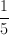of her stock of apples.  On Saturday, she sells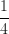of the remaining apples.  What fraction of her original stock of apples does Maria have left for sale on Sunday?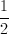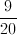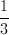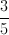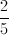Explanation:

Friday:apples sold

Saturday:  This means that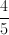of her stock is remaining at this point.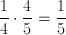of her stock of apples was sold on Saturday.

By the end of Saturday,of her stock has been sold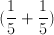.  Ifof her stock has been sold,remains to be sold on Sunday.

### Example Question #1 : Whole And Part

Michael Phelps’ workout program consists of Aerobics, Bench Presses, and Cycling.  Aerobics and Bench Presses take a total of 24 minutes, Bench Press and Cycling take a total of 40 minutes, and the entire workout takes a total of 56 minutes.  How many minutes are required for the Bench Press portion of his workout?

32 minutes

16 minutes

8 minutes

4 minutes

40 minutes

8 minutes

Explanation:

To find your answer, we would use substitution within a system of equations.  If (A+B) +C = 56, we can replace (A+B )= 24 to get (24) + C = 56, Thus making C = 32.  Then we can replace C with 32 in the equation      B+C = 40, thus making B + (32) = 40 to get an answer of 8.

### Example Question #1 : Whole And Part

A wedding planner knows she must deliver 85 small fruits and 40 large fruits to the wedding event. She knows that one basket can hold either 5 small fruits or 3 large fruits. What is the minimum number of baskets she must bring to the event to fulfill their demand?Explanation:

To know the minumum number of baskets required for each size, simply divide the number of fruits required by the basket capacity for each fruit: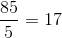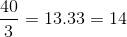(we must round up to nearest whole number for practical purposes)

Since we need to bring both the large and small fruits to the event, we need to add these two values: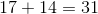baskets total.

### Example Question #422 : Arithmetic

After saving her money for six weeks, Felicia has saved up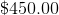. If she saves three-fifths of her pay each week, how much does she make in a week?

Cannot be determined from the information provided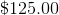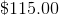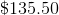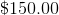Explanation:

When working with fractions or percents, it is often really helpful to write out an equation showing what you need to find. In this case, we know that three-fifths of Felicia's weekly pay (our variable—let's call it) over six weeks results in, so our equation looks like this: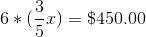So, we want to simplify and rearrange this equation. Start by multiplying thewith the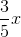: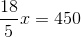Then, multiply both sides by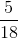, the reciprocal of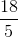: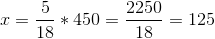So,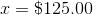, and we now know Felicia's weekly pay!

### Example Question #1 : How To Find The Whole From The Part With Fractions

A company needs 45 pounds of apples to make 30 containers of apple juice. How many pounds of apples would the company need to make 20 containers of apple juice?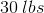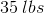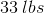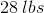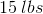Explanation:

To answer this question, we need to set up a proportion for how many pounds of apples it takes to make a certain number of containers, knowing that 45 pounds of apples makes 30 containers of apple juice. We want to figure out how many pounds of apples it would take to make 20 containers of apple juice.

To set up a proportion, we must put the amounts that correspond to each other in the same fraction and equate it to a similar fraction. In this case, we will set up fractions that show containers of apple juice over pounds of apples. So for this data: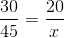Note that we put both of the values for containers of apple juice in the numerator of the fractions and pounds of apples in the denominator. Here,is the value we are looking for.

We then cross-multiply by taking the denominator of each fraction and multiplying each side of the equation by it so that we can create an equation that is solved easier.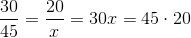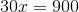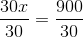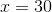Therefore, it takespounds of apples to makecontainers of apple juice.

### All ACT Math Resources